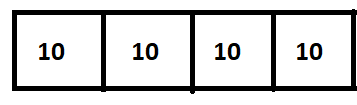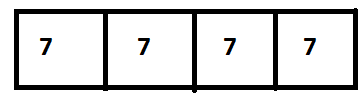# Math in Focus Grade 2 Chapter 16 Practice 2 Answer Key Real-World Problems: Division

This handy Math in Focus Grade 2 Workbook Answer Key Chapter 16 Practice 2 Real-World Problems: Division detailed solutions for the textbook questions.

## Math in Focus Grade 2 Chapter 16 Practice 2 Answer Key Real-World Problems: Division

Circle the correct bar model.

Example

Divide 15 children into 5 groups. How many children are in each group?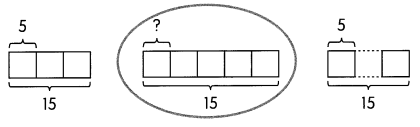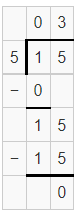Therefore, there are 3 children in each group.

Question 1.
Place 20 strawberries equally on 4- plates. How many strawberries are on each plate?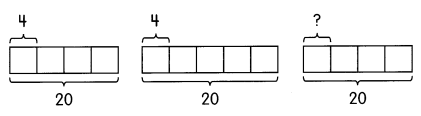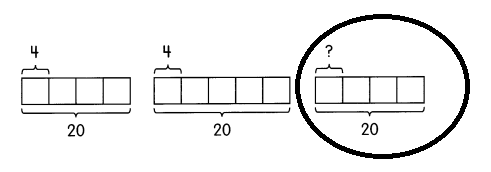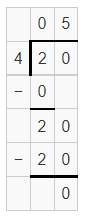Therefore, there are 5 strawberries on each plate.

Question 2.
There are 21 buttons to sew on some shirts. Each shirt needs 3 buttons. How many shirts are there?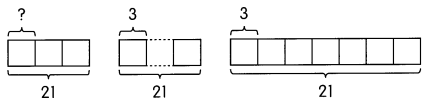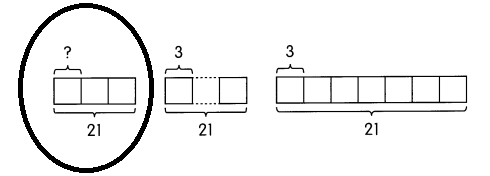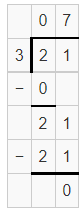Therefore, there are 7 shirts.

Example
A baker has 12 rolls. He divides the rolls equally among 4 children. How many rolls does each child get?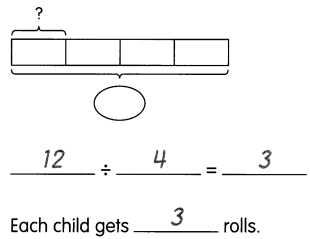Question 3.
Zach has 36 plants and 4 pots. He puts an equal number of plants in each pot. How many plants are in each pot?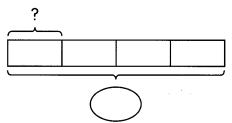__________ ÷ _________ = ____________
There are _________ plants in each pot.
36 ÷ 4 = 9
There are 9 plants in each pot.

Example
Ben has 35 leather strips. He uses 5 strips for each necklace he makes. How many necklaces does he make?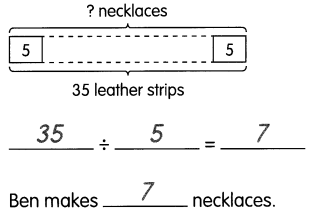Question 4.
Lily sews 24 dresses for her dolls. Each doll gets 3 dresses. How many dolls does Lily have?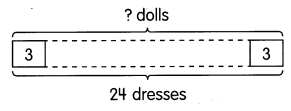__________ ÷ _________ = ____________
Lily has _________ dolls.
24 ÷ 3 = 8
Lily has 8 dolls.

Question 5.
Gina has 40 stamps. She pastes them equally on 4- pages in her album. How many stamps are on each page?
There are __________ stamps on each page.